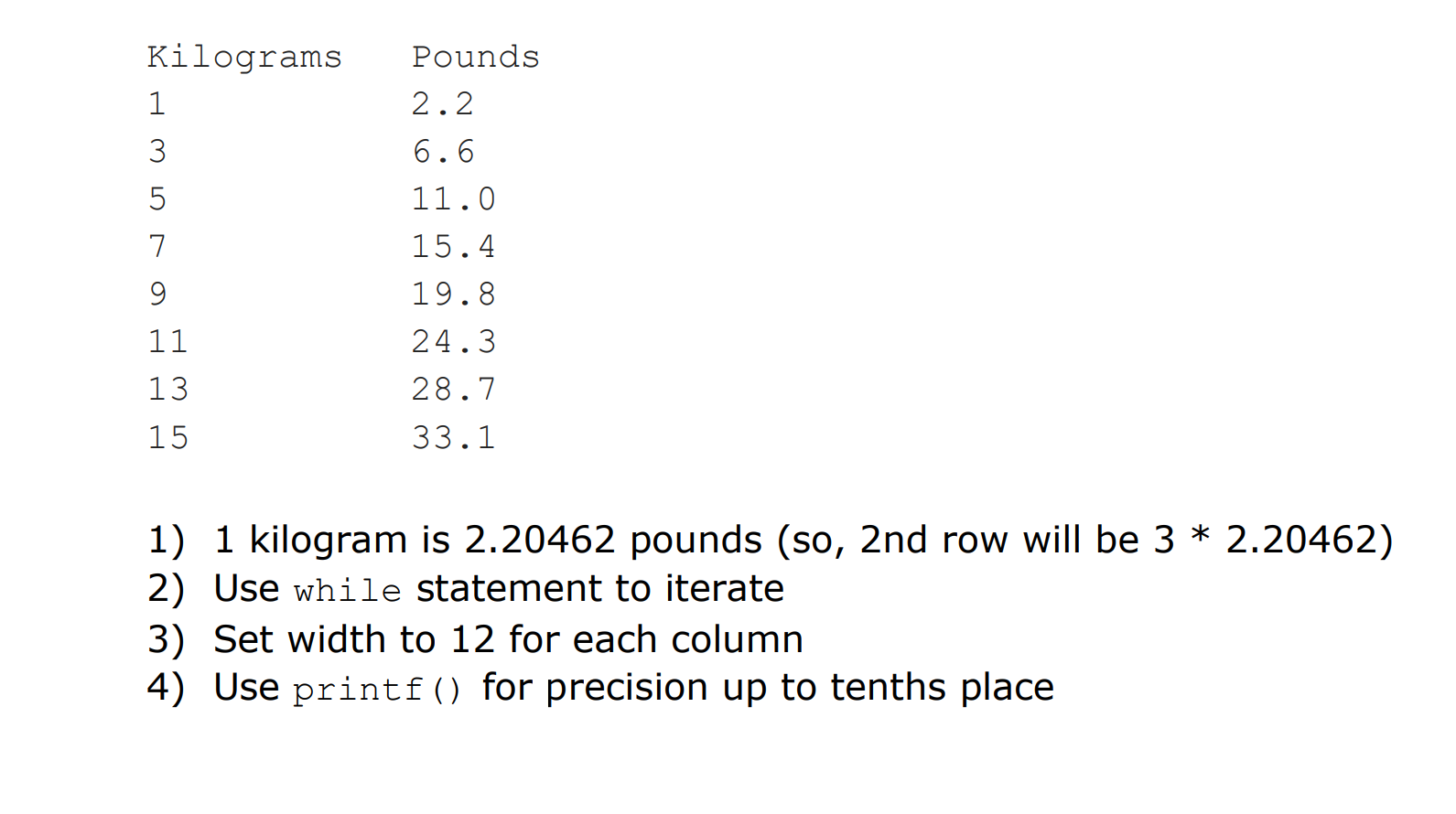# Kilograms Pounds2.26.611.015.419.824.328.733.1713151) 1 kilogram is 2.20462 pounds (so, 2nd row will be 3 * 2.20462)2) Use while statement to iterate3) Set width to 12 for each columrn4) Use printf) for precision up to tenths place

Question
1 views

1. What is the output of the following code?
public class Loopy {
public static void main(String[] args) {
int x = 1;
System.out.println("Before the loop");
while (x < 4) {
System.out.println("In the loop");
System.out.println("Value of x is " + x);
x = x + 1;
}
System.out.println("This is after the loop");
}
}

2. Write a program called KiloToPoundTable that prints the below table. Use
printf()and while statement. Do not use if while statement.

1) 1 kilogram is 2.20462 pounds (so, 2nd row will be 3 * 2.20462)
2) Use while statement to iterate
3) Set width to 12 for each column
4) Use printf() for precision up to tenths placehelp_outlineImage TranscriptioncloseKilograms Pounds 2.2 6.6 11.0 15.4 19.8 24.3 28.7 33.1 7 13 15 1) 1 kilogram is 2.20462 pounds (so, 2nd row will be 3 * 2.20462) 2) Use while statement to iterate 3) Set width to 12 for each columrn 4) Use printf) for precision up to tenths place fullscreen
check_circle

Step 1

1. Algorithm for the given code is as follows:

The variable ""x is initialized as "1" within the "main()" function.

If the variable "x" is equal to "1" then it print "Before the loop".

If the variable "x" is less than "4" then it print "In the loop".

If the variable "x" is greater than "4" then it print "This is after the loop".

Step 2

Output of the given program is as follows:

Step 3

2. The program has the following algorithm:

1. The variables “pound”, and “kilo” is initialized within the “main()” function.
2. The “while” statemen...

### Want to see the full answer?

See Solution

#### Want to see this answer and more?

Solutions are written by subject experts who are available 24/7. Questions are typically answered within 1 hour.*

See Solution
*Response times may vary by subject and question.
Tagged in

### Computer Science